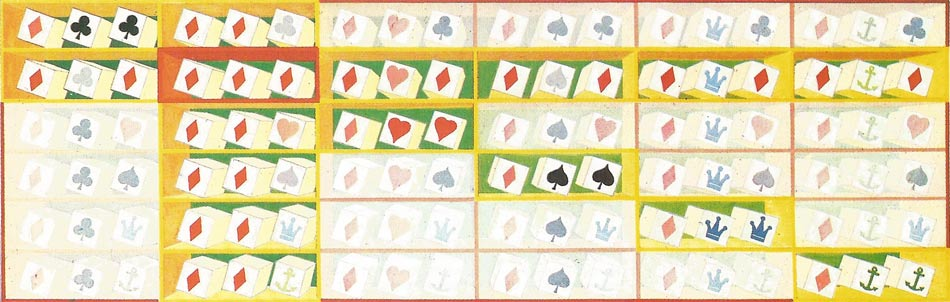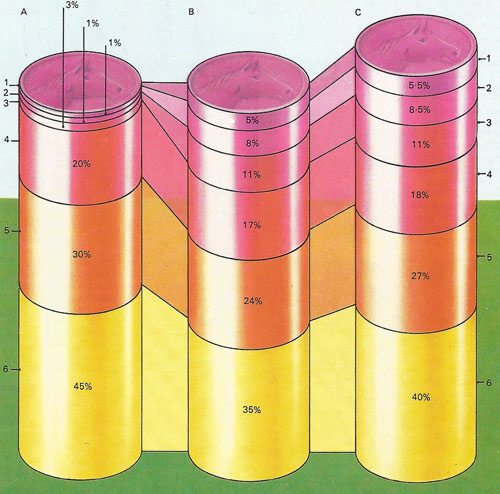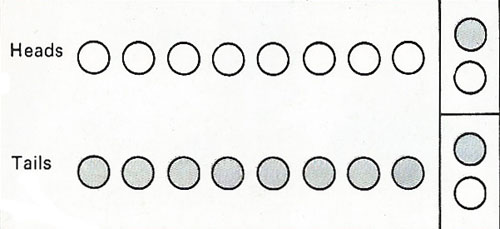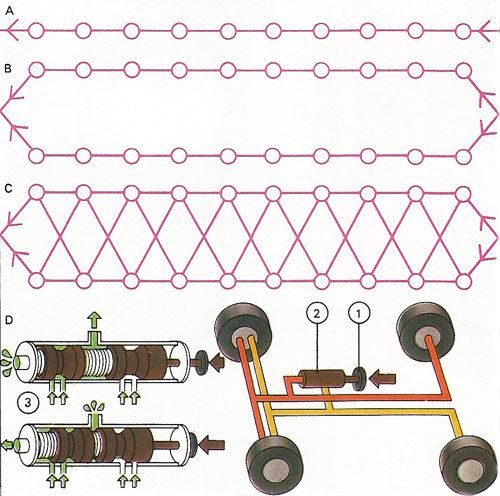A# probabilityIs the rational grid layout of Salt Lake City [A] more efficient than the rambling European city of Cracow [B]? A diagonal journey on a grid forces you to traverse the equivalent of two sides of a triangle, even if you zigzag. Probability theory shows that to facilitate many unpredictable point-to-point journeys, a random distribution of straight lines is best [C], a style close to Cracow's.Crown and anchor uses three dice inscribed with the six symbols of the matrix below, which shows all outcomes for the first die "diamond" (five other matrices are similar). Players bet on their symbols against a banker, who returns twice the stake for one symbol displayed, three times for double and four times for triple. Assume each symbol is backed, giving six stakes "input" per throw. 20 out of 36 times (by the matrix), three different symbols come up and the banker makes no gain, returning three 2-fold stakes to the winners. On doubles (15 in 36) he pays out two on the singlet and three on the doublet and keeps one. On the only triple, he pays four and keeps two. So in 36 rounds he has gained (with one unit staked on each symbol) 15+2=17 of the 216 stakes: 7.9% return.The bookmaker aims to offer odds that give him the same predictable profits whichever horse wins. Thus if he received \$3 on one horse and \$5 on another, he might offer odds of 7:3 on the first (4 to 3 on) and 7:5 (2 to 5 on) on the second. Whichever wins, he pays out \$7 and makes \$1 profit. So his odds reflect the money bet. "Outsiders" attract little money, so he offers long odds on them. The chances of six horses are shown in A; 1,2,3 are outsiders with very long odds against them. But novice betters find them unreasonably seductive, so the money placed distributes itself as in B. The bookmaker changes the total odds upwards in his own favor, as in C. He is sure of a profit in the ratio C to B. But even so, some winning odds - on the favorite  - are undervalued in C compared to the "reality" of A: 40% (i.e. offering a return of 100 to 40) compared to its actual chance of winning, 45%. Hence 6 favors the punter and a series of such bets should clear an average of 10% profit to him. But the gullible backers of outsiders, in the long run, also lose. The same mathematical calculation of odds - probabilities - occurs throughout science. In atomic theory, for example, the location of an electron within an atom is defined in terms of probabilities.A tossed coin can land either "heads" or "tails". On each toss the probability Is the of a head (or tail) is 1/2 (0.5) - the chances are evens. If a coin lands heads (or tails) eight times in succession, a gambler might be tempted to expect that a tail (or head) is more likely to occur on the ninth toss. But the mathematical probability of either outcome is still exactly 1/2 - an even chance.Dice and coin tossing.A chain of components, all of which must work if the system is to function, is less reliable than its members.

Probability is the likelihood that a given event will occur expressed as the ratio of the number of actual occurrences, n, to the number of possible occurrences, N: n/N; where all of N are equally likely. For example, when throwing a die there is 1 way in which a six can turn up and 5 ways in which a "not six" can occur. Thus n = 1 and N = 5 + 1 = 6, and the ratio n/N = 1/6. If two dice are thrown there are 6 × 6 (= 36) possible pairs of numbers that can turn up: the chance of throwing two sixes is 1/36. This does not mean that if a six has just been thrown there is only a 1/36 chance of throwing another: the two events are independent; the probability of their occurring together is 1/36.

## More on calculating probabilities

This is an elementary but popular fallacy about probability theory. If two independent events each have a known probability such as one-thousandth, the chance of their both occurring together is indeed obtained by multiplying the two probabilities giving in the example one-millionth. But they must be independent: the chance of one cannot be altered by tampering with that of the other – such as ensuring its certainty.

This multiplication rule is one of the two great pillars of probability theory. The other, the addition rule, says that given two mutually exclusive events (such as rolling a one or two with a dice – both cannot be rolled), then the chance of either occurring is the sum of their probabilities. In this case each has a 1/6 probability, so if either one or two wins, the chance of success is 1/6 = 1/6 = 1/3.

These two rules, carefully used, can solve most problems of probability. They rest on a subtle sort of probabilistic "atomic theory" that takes any chance event as being compounded from a set of basic "equiprobable events". By calculating what combination of these will result in the desired chance coming up, its probability is obtained. But the notion requires subtle handling. Many misleading arguments depend on a deceptive choice of basic equiprobabilities. What is the chance of there being monkeys on Mars, for example? Either there are or are not – and it could be argued that, since nobody has yet been to Mars, these mutually exclusive situations are equally probable. Then each has half a chance of truth and there is a 50 per cent chance that there are monkeys on Mars.

More subtly, what is the chance of getting one head and one tail on two tosses of a coin? It might be reasoned that there are only three basic probabilities: two heads, head and tail, and two tails. Only one of these is favorable, so the chance is 1/3. But this is not so. There are actually four "atomic" equiprobabilities: HH, HT, TH and TT (where H stands for heads and T stands for tails), of which two are favorable. The chance is 2/4, or one half.

## Chances of success

In mathematical notation, chances vary from 0 (impossible) to 1 (certain). If there are 7 equiprobable possibilities, and 2 of them will result in success, the chance of success is 2 in 7, or 2/7, or 0.2857. This can also be expressed as 28.57 per cent, or in betting parlance 2 to 5 on, or 5 to 2 against. Such figures make most intuitive sense when applied to situations that can occur many times. In a run of 7,000 trials each with a 2/7 chance of success, about 2,000 successes would be expected. A gambler would break even in the long run by accepting odds of 7 to 2 (that is \$7 return for a \$2 stake). Where the basic equiprobable events are clear and knowable (as in the fall of coins, dice or cards), probability theory can give unambiguous chances of success for any outcome. All casinos and gambling houses use this principle to set fixed odds that give them a small advantage.

In sports and business assessments, odds are subjective and different people guess them differently. By betting on the favorite in a horse-race with a number of unproven "outsiders", however, the gambler's chances of winning are demonstrably better. If one of the horses is known to be doped, or a rival's business strategy is known, it is possible to place investments with better-than-average insight. This is the province of game theory - the theory of competing for gains against opponents who possess assumed aims and knowledge.

In the child's game of button-button a button is hidden in one hand and the opponent has to guess which. He wins a penny if he is correct and loses one if he is wrong. What is the best strategy for the holder? If the same hand is always played, or hands are switched regularly, the opponent will soon outguess the holder. Game theory proves that the best strategy is to decide the switch at random, for example by tossing a coin before each round. This is entirely foolproof; even if the opponent discovers the strategy he cannot win more than he loses in the long run. But if two pennies are lost for a right-hand disclosure and only one penny for a left-hand one, the opponent could then win steadily by always choosing the right hand, and making on average bigger gains than losses. For this modification, game theory prescribes for the holder "weighted random switch" of 2:1 towards the left – say by tossing a die and playing to the right on 1 and 2, but to the left on 3, 4, 5 and 6.

## Permutations and combinations

Consider throwing a die six times with the aim of getting each of the numbers exactly once. If you want to do this in order (a permutation), say from 1 to 6, working out the probability of your doing so is easy: you have 1/6 chance of throwing a one, 1/6 chance of throwing a two, and so on; so that the probability of a favorable result overall is (1/6)6 = 1/46656. If you are not concerned with the order (combination) the situation is different: the probability of a favorable result on the first throw is 1 (any number is favorable), on the second 5/6, and so on, so the overall probability is

6/6 × 5/6 × 4/6 × 3/6 × 2/6 × 1/66

or 6!/66 (see factorial) – 1/65. Clearly one is more likely to succeed with a desired combination than with a permutation.

## Applications of probability

Probability theory is plainly intimately linked with statistics. More advanced probability theory has contributed vital understandings in many fields of physics, as in thermodynamics, behavior of particles in a colloid (see Brownian motion), or molecules in a gas, and atomic physics.

In real-life conflicts such as war and business, game theory is often used for clarifying options, but seldom slavishly followed. If two people make an agreement, for example, game theory recommends to each that he double-crosses the other, for he will gain more if the other is honest. And in a world of unique events that either happen or do not, the whole concept of probability needs careful handling. Be warned by Peter Sellers parody of a politician, who "does not consider present conditions likely".